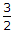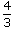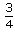# Civil Engineering - UPSC Civil Service Exam Questions

6.

What is the ratio of maximum shear stress to average shear stress for a circular section ?

 A. 2 B.C.D.Explanation:

No answer description available for this question. Let us discuss.

7.

If a circular shaft is subjected to a torque T and a bending moment 'M' the ratio of the maximum shear stress to the maximum bending stress is given by :

 A. 2M/T B. T/2M C. 2T/M D. M/2T

Explanation:

No answer description available for this question. Let us discuss.

8.

Which one of the following statements is not correct ?

 A. Models are always smaller than the prototypes. B. Dynamic similarity between a model and a prototype can be verified by equating Reynold's number in a viscous flow. C. Mach number achieves significance when the velocity of fluid approaches or exceeds the sonic velocity. D. Distorted models are always exaggerated on a vertical scale.

Explanation:

No answer description available for this question. Let us discuss.

9.

The construction of impounding reservoir is required when

 A. average annual flow in the stream is lower than average demand B. the rate of flow in the stream, in dry season in more than the demand C. the rate of flow in the stream, in dry season is less than the demand D. the rate of flow in the stream is equal to the demand

Explanation:

No answer description available for this question. Let us discuss.

10.

The method of plane tabling commonly used for establishing the instrument station is a method of

 A. radiation B. intersection C. resection D. traversing